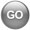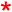Configure Sentronic HD high-definition Pressure Regulator
ASCO Numatics Sentronic HD high-definition Pressure Regulator Configurator
 Enter valid model number or use configurator below to generate a valid number.Please Configure a model number using the attributes belowIndicates a required attributes. 0 of 0 required attributes selected.
Model Number Generator
 G 616 A
 Pipe Size 4 = G1/4 + Pressure hold 5 = G1/4 + Pressure release

 Setpoint 0 = 0-10 V 1 = 0-20 mA 2 = 4-20 mA 3 = PWM - Frequency

 Feedback 0 = 0-10 V 1 = 0-20 mA 2 = 4-20 mA

 Output 2 0 = NC 1 = Digital OUT 2 = Analog OUT 2

 Input 2 / Display with buttons 0 = Standard NC + Display 2 = Analog IN 2 + Display 4 = Digital IN 2 + Display 6 = Frequency IN + Display *

 Pressure Range 03 = 0...3 bar 06 = 0...6 bar 10 = 0...10 bar V1 = -1 ... (+3 bar

 Option A00 = Standard